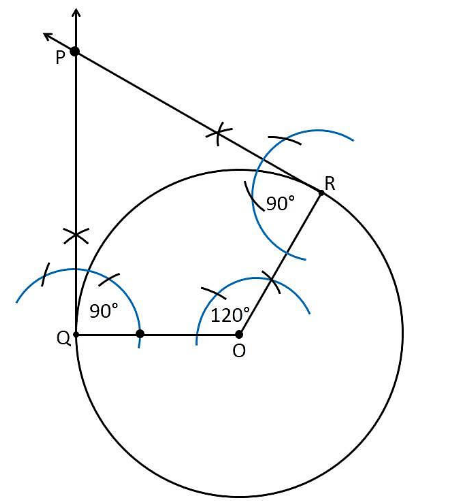Guru

# Draw a pair of tangents to a circle of radius 5 cm which are inclined to each other at an angle of 60° Q.4

• 0

What is the best and the simple way to solve the problem of question 4 of Constructions of exercise 11.2 of class 10th math, suggest me the simple way to solve this problem Draw a pair of tangents to a circle of radius 5 cm which are inclined to each other at an angle of 60°

Share

1. Construction Procedure:

The tangents can be constructed in the following manner:

1. Draw a circle of radius 5 cm and with centre as O.

2. Take a point Q on the circumference of the circle and join OQ.

3. Draw a perpendicular to QP at point Q.

4. Draw a radius OR, making an angle of 120° i.e(180°−60°) with OQ.

5. Draw a perpendicular to RP at point R.

6. Now both the perpendiculars intersect at point P.

7. Therefore, PQ and PR are the required tangents at an angle of 60°.Justification:

The construction can be justified by proving that ∠QPR = 60°

By our construction

∠OQP = 90°

∠ORP = 90°

And ∠QOR = 120°

We know that the sum of all interior angles of a quadrilateral = 360°

∠OQP+∠QOR + ∠ORP +∠QPR = 360o

90°+120°+90°+∠QPR = 360°

Therefore, ∠QPR = 60°

Hence Justified

• 0